# Properties of Soil Formula – Soil Mechanics

#### 1. Water Content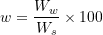where ,

Ww = Weight of water

Ws = Weight of solids

Note – There can be no upper limit to water content i.e. w≥0

#### 2. Void Ratio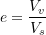where ,

Vv = Volume of voids

Vs = Volume of solids

Note – though size of individual void of coarse grained soil is more , total volume of voids in fine grained soil is more

in general case there is no upper limit for void ratio . i.e. e>0

#### 3. Porosity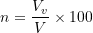where ,

Vv = Volume of voids

V = Total Volume of soil

Note – 1. Porosity cannot exceed 100% i.e. 0<n<100

2. in comparison to porosity , void ratio is more frequently used because volume of solids remains same , whereas total volume changes

#### 4. Degree of Saturation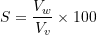where ,

Vw = Volume of water

Vv = Volume of voids

Note – 1. Range of Degree of saturation. 0≤S≤100

2. For perfectly dry soil – S=0

3. For Fully saturated soil – S=100%

#### 4. Air Content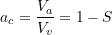where ,

Va = Volume of air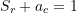where S= Degree of saturation

#### 5. Percentage Air Void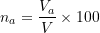where ,

Va = Volume of air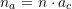#### 5. Unit Weight

##### (A) Bulk unit Weight (γ)

Bulk unit weight is total weight per unit volume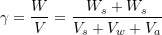##### (B) Dry Unit Weight (γd)

Dry unit weight is the weight of soil solids per unit volume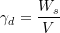Note – Dry unit weight is used as a measure of denseness of soil . more dry unit weight means more compacted soil

##### (C) Saturated Unit weight (γsat)

Saturated unit weight is the ratio of total weight of fully saturated soil sample to its total volume.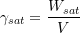##### (C) Submerged Unit weight or Buoyant unit weight (γ′)

Submerged  unit weight is the ratio of submerged weight of  soil solid per unit volume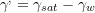Updated: January 9, 2023 — 10:44 pm